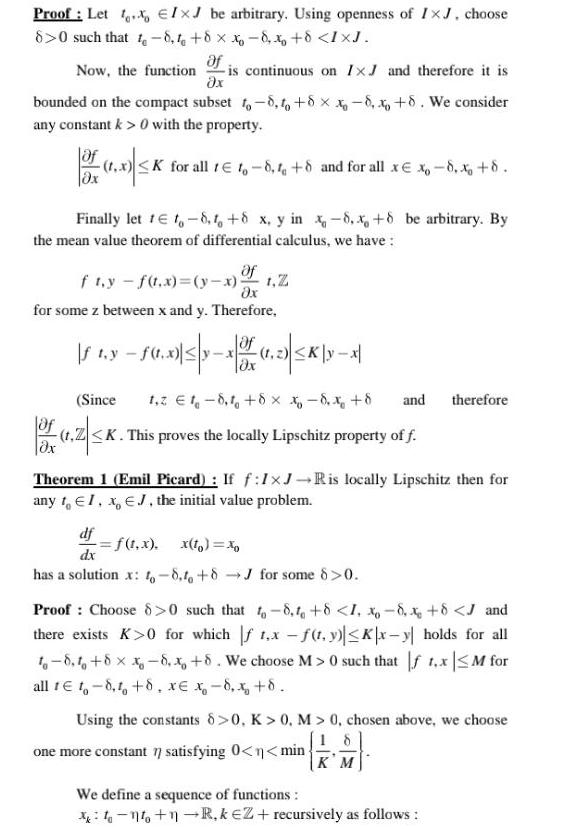Question:

# Proof Let Ix be arbitrary Using openness of IxJ choose 8 0

Last updated: 9/18/2023Proof Let Ix be arbitrary Using openness of IxJ choose 8 0 such that t 8 1 8 x 8 x 8 IXJ Of Now the function is continuous on IxJ and therefore it is x bounded on the compact subset to 8 to 8 x 8 x 8 We consider any constant k 0 with the property 1 0 K K for all tto 8 1 8 and for all xx 8 x 8 x Finally let 1 t 8 1 8 x y in x 8 x 8 be arbitrary By the mean value theorem of differential calculus we have Of fty f t x y x 1 Z x for some z between x and y Therefore 1 350 3053 1 1 2 K yx If t y f t x z Ox Since 1 2 18 1 8 x x 8 x 8 and therefore of 1 Z K t Z K This proves the locally Lipschitz property of f dx Theorem 1 Emil Picard If f 1 J Ris locally Lipschitz then for any to EI x EJ the initial value problem df f t x x to dx has a solution x 8 to 8J for some 8 0 Proof Choose 8 0 such that to 8 1 8 1 8 x 8 J and there exists K 0 for which f 1 x f t y Kx y holds for all t 8 8 x 8 x 8 We choose M 0 such that f 1 x M for all te t 8 1 8 x x 8 x 8 Using the constants 8 0 K 0 M 0 chosen above we choose 18 one more constant 7 satisfying 0 n min KM We define a sequence of functions 1 1 R keZ recursively as follows